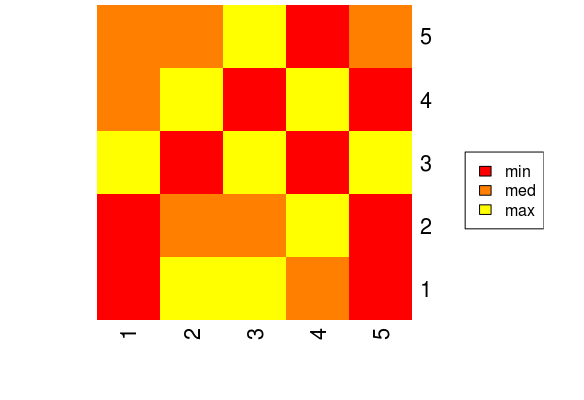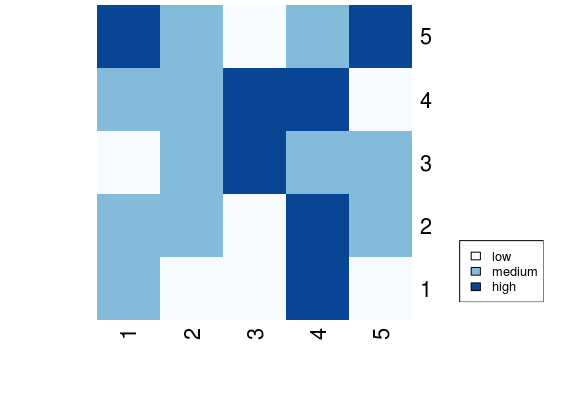# How to show legend in heatmap in R?

• Last Updated : 23 Feb, 2021

A heat map is a graphical representation of data where each data value is represented in terms of color value. Heatmap is created using heatmap() function in R. Legend associated with histogram makes it easy to understand what the color values mean. Legend is shown with histogram using legend() function in R.

### Function Used

• heatmap() function in R Language is used to plot a heatmap. Heatmap is defined as a graphical representation of data using colors to visualize the value of the matrix.

Syntax: heatmap(data)

Parameters:
data: It represent matrix data, such as values of rows and columns

Return: This function draws a heatmap

• legend() function in R Language is used to add legends to an existing Plot. A legend is defined as an area of the graph plot describing each of the parts of the plot. The legend plot is used to show statistical data in graphical form.

Syntax:
legend(x, y, legend, fill, col, bg, lty, cex, title, text.font, bg)

Parameters:
x and y: These are co-ordinates to be used to position the legend
legend: Text of the legend
fill: Colors to use for filling the boxes with legend text
col: Colors of lines
bg: It defines background color for the legend box.
title: Legend title (optional)
text.font: An integer specifying the font style of the legend (optional)

Returns: Legend plot

### Approach

• Create data matrix
• Plot heatmap using heatmap() method
• Provide appropriate attributes with respective values
• Associate legend with heatmap using legend() method
• Run code to display plot

Example 1:

## R

 `# Create data matrix``A <- ``matrix``(``rnorm``(25, 0, 5), nrow = 5, ncol = 5)  ``print``(A)`` ` `# Plot a heatmap ``heatmap``(A,Rowv=``NA``,Colv=``NA``,col=``heat.colors``(3))`` ` `# Plot a corresponding legend``legend``(x=``"right"``, legend=``c``(``"min"``, ``"med"``, ``"max"``),fill=``heat.colors``(3))`

Output:Example 2:

## R

 `# Import library for color``library``(RColorBrewer)`` ` `# Create data matrix``A = ``matrix``(``  ``c``(1,2,0,3,4,0,2,1,3,3,0,0,4,``    ``4,1,4,3,1,4,3,0,2,1,1,4),``  ``nrow = 5, ncol = 5)        `` ` `# Plot a heatmap``heatmap``(A, Rowv = ``NA``, Colv = ``NA``, ``        ``col = ``colorRampPalette``(``brewer.pal``(8,``"Blues"``))(3))`` ` `# Plot a legend in bottom right part of heatmap``legend``(x = ``"bottomright"``, legend = ``c``(``"low"``, ``"medium"``, ``"high"``),``       ``cex = 0.8, fill = ``colorRampPalette``(``brewer.pal``(8, ``"Blues"``))(3))`

Output:My Personal Notes arrow_drop_up